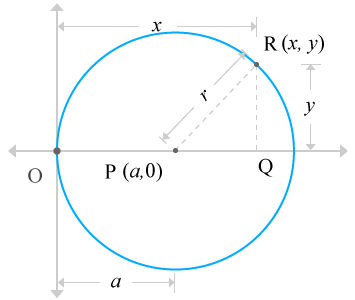# Equation of the Circle when circle passes through the origin and centre of the circle lies on x-axis

Expressing a circle in a mathematical form expression when the circle passes through the origin of Cartesian coordinate system and centre of the circle lies on $x$-axis is defined Equation of the Circle when circle passes through the origin and centre of the circle lies on $x$-axis.

Geometrically, a circle can be passed through the origin of the Cartesian coordinate geometric system and also the centre of the same circle may also lie on the horizontal x-axis. It can be expressed in a mathematical equation form in mathematics by understanding the relation of circle with Cartesian coordinate system and it understands us how exactly a circle is, in Cartesian coordinate system.

## Derivation

Consider a circle, whose radius is assumed as $r$ units. Also assumed, the centre of the circle lies on the $x$-axis and the circle passes through the origin of the Cartesian coordinate system.Assume, the centre of the circle is denoted by a letter $P$ and the point $P$ is located at $a$ units distance from the origin in horizontal $x$-axis direction but the point is lied on the $x$-axis. So, the vertical distance of this point $P$ is zero units. Therefore, the coordinates of the point $P$ is $P\left(a,0\right)$.

Consider a point on the circle, called point $R$ which is located at $R\left(x,y\right)$. Assume, it is a variable point, which represents each point on the circle.

Draw a line towards horizontal axis from the point $R$ perpendicularly and draw a line from point $P$ on the horizontal axis until both lines get intersected at a point, called $Q$. This procedure constructed a right angled triangle, known $\Delta RPQ$.

The triangle is a right angled triangle. So, applying Pythagorean Theorem to triangle $\Delta RPQ$ gives us relation between three sides of the triangle.

${PR}^{2}={PQ}^{2}+{RQ}^{2}$

The values of the each side of the right angled triangle can be evaluated from the right angled triangle $\Delta RPQ$.

• Length of the Opposite side is $RQ=y$
• Length of the Adjacent side is
• Length of the Hypotenuse is $PR=r$

Substitute these three values in the mathematical relation, developed from the Pythagorean Theorem.

The point $P$ is located at $a$ units distance from the origin on the $x$-axis. So, the length of the $OP$ is equal to $a$ units; means $OP=a$. Actually, the point $P$ is centre of the circle and the circle passes through the origin. So, the distance from origin $O$ to point $P$ is known radius of the circle. It means $OP=r$. Therefore, $OP=r=a$. As per this rule, the algebraic expression can be written as follows.

It’s an algebraic equation, which represents a circle when the circle is passing through the origin and also its centre lies on the $x$-axis.

Latest Math Topics
Latest Math Problems
##### Recommended Math Concepts###### How to derive equation of a Circle when the circle passes through the origin###### How to develop equation of a Circle when the Circle touches x-axis###### How to derive equation of a Circle when the Circle touches y-axis###### How to develop equation of a Circle when the Circle touches both axes###### How to derive Equation of the Circle when centre of the circle coincides with the origin###### How to derive Equation of a Circle when Circle passes through the origin and Centre of the Circle lies on y-axis###### How to develop Equation of the Circle when Circle doesn’t passes through the origin and Centre of the Circle lies on x-axis###### How to develop Equation of the Circle when Circle doesn’t passes through the origin and Centre of the Circle lies on y-axis###### How to develop Equation of a Circle in Standard form

A best free mathematics education website for students, teachers and researchers.

###### Maths Topics

Learn each topic of the mathematics easily with understandable proofs and visual animation graphics.

###### Maths Problems

Learn how to solve the maths problems in different methods with understandable steps.

Learn solutions

###### Subscribe us

You can get the latest updates from us by following to our official page of Math Doubts in one of your favourite social media sites.